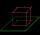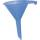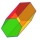# Problems of the area of a right triangle

#### Number of problems found: 508Find the height and surface of a regular quadrilateral pyramid with a base edge a = 8cm and a wall height w = 10cm. Sketch a picture.
• Hexa pyramidThe base of the regular pyramid is a hexagon, which can be described by a circle with a radius of 1 m. Find the volume of the pyramid 2.5 m high.
• Triangular prismCalculate a triangular prism if it has a rectangular triangle base with a = 4cm and hypotenuse c = 50mm and height of the prism is 0.12 dm.
• Hexa prismDetermine the volume of hex prism with edge base 4 cm. The body height is 28 cm.
• Cone roofHow many m2 of roofing is needed to cover a cone-shaped roof with a diameter of 10 m and a height of 4 m? Add an extra 4% to the overlays.
• Tetrahedral pyramidCalculate the surface S and the volume V of a regular tetrahedral pyramid with the base side a = 5 m and a body height of 14 m.
• Body diagonalFind the cube surface if its body diagonal has a size of 6 cm.
• Tetrahedral pyramidWhat is the surface of a regular tetrahedral (four-sided) pyramid if the base edge a=16 and height v=16?
• Surface of pyramidIn a regular quadrilateral pyramid, the height of the sidewall is equal to the length of the edge of the base. The content of the sidewall is 32 cm2. What is the surface of the pyramid?
• 3s prismIt is given a regular perpendicular triangular prism with a height of 19.0 cm and a base edge of 7.1 cm. Calculate the volume of the prism.
• SailsWe known heights 220, 165, and 132 of sail. It has a triangular shape. What is the surface of the sail?
• Surface area 6Find the surface area of a prism whose bases are right triangles with sides of length 3, 4, and 5 inches and a height of 8 inches. Include a sketch
• How toHow to find a total surface of a rectangular pyramid if each face is to be 8 dm high and the base is 10 dm by 6 dm.
• Pyramid heightFind the volume of a regular triangular pyramid with edge length a = 12cm and pyramid height h = 20cm.
• Cube cutThe cube ABCDA'B'C'D ' has an edge of 12cm. Calculate the area of diagonal cut B DD'B '.
• FunnelThe funnel has the shape of an equilateral cone. Calculate the surface wetted with water if we poured into the funnel 7.1 liters of water.
• HexagonCalculate the regular hexagonal prism's surface whose base edge a = 12cm and side edge b = 3 dm.
• The volumeThe volume of the cone is 94.2 dm³, the radius of the base is 6 dm. Calculate the surface of the cone.
• Sphere cutsAt what distance from the center intersects sphere with radius R = 56 plane, if the cut area and area of the main sphere circle is in ratio 1/2.
• Above EarthTo what height must a boy be raised above the earth to see one-fifth of its surface.

Do you have an exciting math question or word problem that you can't solve? Ask a question or post a math problem, and we can try to solve it.

We will send a solution to your e-mail address. Solved examples are also published here. Please enter the e-mail correctly and check whether you don't have a full mailbox.

See also our right triangle calculator. Right triangle Problems. Area - math word problems.